# Line Segment

In geometry, a line segment is bounded by two distinct points on a line. Or we can say a line segment is part of the line that connects two points. A line has no endpoints and extends infinitely in both the direction but a line segment has two fixed or definite endpoints. The difference between a Line segment and a ray is that a ray has only one endpoint and its other end extends infinitely.

A one-dimensional shape and collection of points extending infinitely in either direction is a line. The below figure shows a line.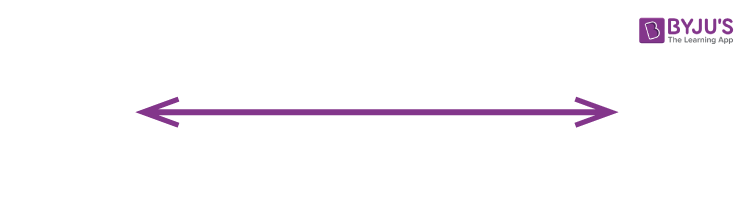Line

A part of a line that has two endpoints and is the shortest distance between them, is a line segment. The below figure shows a line segment.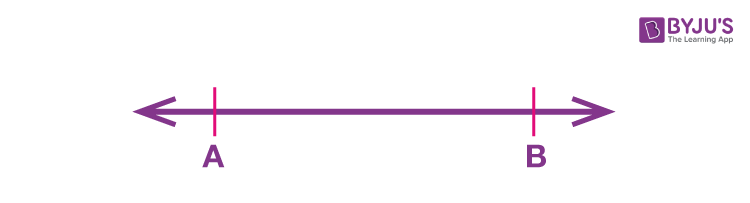Line Segment

A ray begins at one endpoint and ends at infinity. See the below figure of a ray.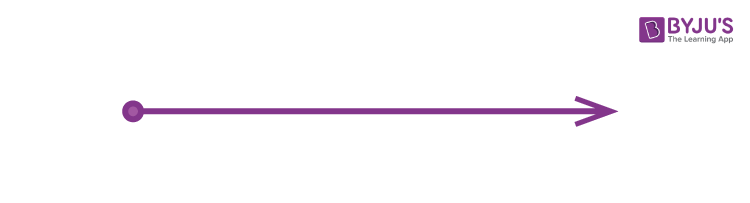Ray

## Line Segment Definition

A line segment has two definite endpoints in a line. The length of the line segment is fixed, which is the distance between two fixed points. The length here can be measured by metric units such as centimeters (cm), millimeters (mm) or by conventional units like feet or inches.  While learning the concept of lines and angles in Class 7, you will come across the basic terminologies related to it. But in Class 9 the advanced version will be described.

A closed line segment consists of both endpoints, whereas an open line segment is exclusive of the two endpoints. A line segment that has exactly one endpoint is called a half-open line segment.

### Symbol

• $$\begin{array}{l}\text{A line segment with two endpoints A and B and is denoted by the bar symbol, such as } \overline{AB}.\end{array}$$
• $$\begin{array}{l}\text{A line is usually represented by }\leftrightarrow.\end{array}$$
• $$\begin{array}{l}\text{A ray by a right arrow }\rightarrow.\end{array}$$

## Line Segment Formula

As we know, a line segment has two endpoints. Now if we know the coordinates of the endpoints, then we can calculate the length of the line segment by distance formula.

\begin{array}{l}\begin{aligned} &D=\sqrt{\left(x_{2}-x_{1}\right)^{2}+\left(y_{2}-y_{1}\right)^{2}} \end{aligned}\end{array}

Suppose a line segment has coordinates (2, –3) and (–1, –2). Find the length of line segment.

Therefore, by distance formula,

\begin{array}{l}\begin{aligned} D &=\sqrt{(2-(-1))^{2}+(-3-(-2))^{2}} \\ &=\sqrt{(2+1)^{2}+(-3+2)^{2}} \\ &=\sqrt{(3)^{2}+(-1)^{2}} \\ &=\sqrt{9+1}=\sqrt{10} \approx 3.16 \end{aligned}\end{array}

## Measuring Line Segment

How to measure a given line segment? We will learn here through different methods.

### By Observation

The most trivial method of comparison of two line segments is simple observation. Just by observing two line segments, one can predict which is long or short compared to the other.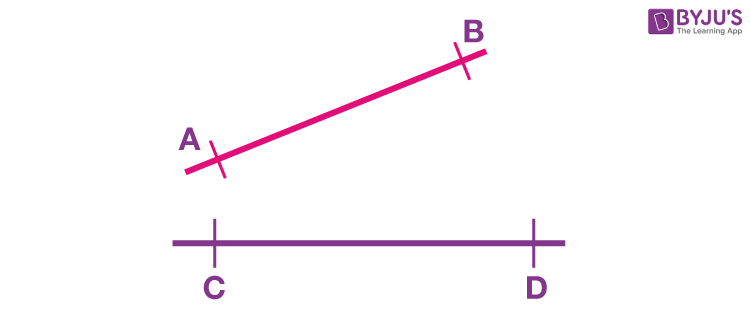In the above figure, by observation itself, we can say that the line segment CD is greater in length as compared to the line segment AB. But this method has several constraints, every time we cannot rely simply on observation to compare two line segments.

### Using Trace Paper

With the help of trace paper, two line segments can be easily compared. Trace one line segment and place it over on the other segment and it can be easily deduced which is greater in length. For more than two line segments repeat the process again and again.

For precise comparison, the line segments must be traced accurately. Hence this method depends on the accuracy of tracing which puts a limitation on this method.

### Using Ruler and Divider

There are certain markings on the ruler beginning from zero as shown in the fig. given below, these markings divide the ruler into equal parts. Each part is equal to a length of 1 cm and these unit centimeters are further subdivided into 10 parts and each sub-part is equivalent to 1 millimeter.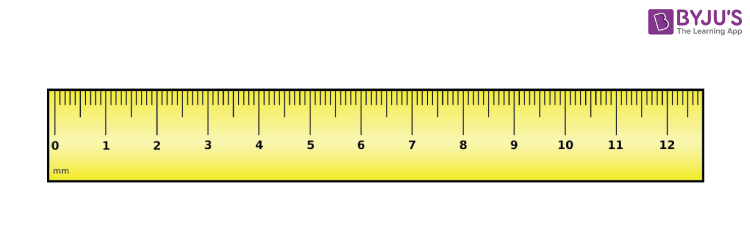To measure a line segment AB, place the zero marking of the ruler along the beginning of the line and measure its length accordingly.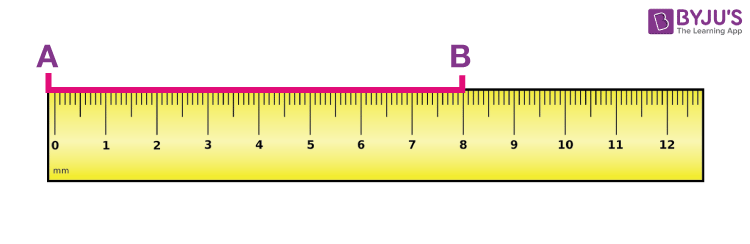In the figure given above, the length of line segment AB is 8 cm.

To eliminate the positioning error we use a divider. Place one of the needles of the divider at A and other at B and then place the divider along the ruler and measure its length. This method is more accurate and reliable.

### Construction of Line Segment

Here we will learn to draw a line segment with the help of a compass and measuring ruler or scale.

• Let us assume, we need to draw a line segment of length 5cm. Then follow the below-given steps:
• Draw a line of any length without a measurement (keeping in consideration of the length of the line segment)
• Mark a point A on the line, which will be the starting point of the line segment.
• Now using a scale or ruler, locate the pointer of the compass 5cm apart from the pencil’s lead.
• Again, place the pointer of the compass at point P on the line and with the same measurement mark an arc with the pencil.
• Now, mark this point as B

Hence, AB is the required line segment of length 5cm.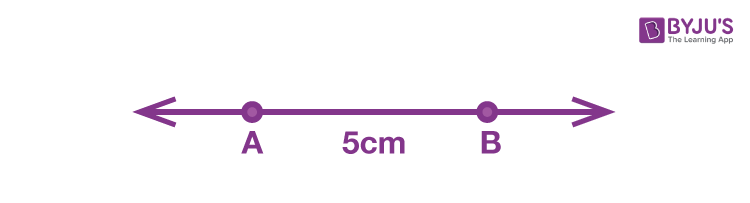## Examples of Line Segments

The most common examples we can see in 2d geometry where all the polygons are made up of line segments.

• A triangle is made up of three line segments joined end to end
• A square is made up of four-line segments
• A pentagon is made up of five-line segments

It is, therefore, line segments play an important role in geometry

### Practice Problems

• Draw a line segment of length equal to 5 cm.
• Draw a line segment of 8.5 cms
• Draw a line segment of 3.5 cms using a ruler and compass

Hence, PQ is the required line segment of length 7cm.

## Frequently Asked Questions – FAQs

Q1

### What is a line segment?

A line segment is a part of line that has two distinct endpoints. These endpoints are definite in nature.
Q2

### How is a line segment different from a line?

A line segment has endpoints whereas a line extends infinitely at both ends.
Q3

### What is the difference between a line segment and a ray?

A ray has only one endpoint whereas a line segment has two endpoints. One end of the ray extends infinitely but the endpoints of a line segment are definite always.
Q4

### What is the symbol of a line segment?

A line segment is denoted by a Bar (-) on the top of its notation, say AB.
Q5

### What are the examples of line segments in real life?

A ruler, a scale, a stick, a boundary line, etc., are examples of the line segment
Quiz on Line segment